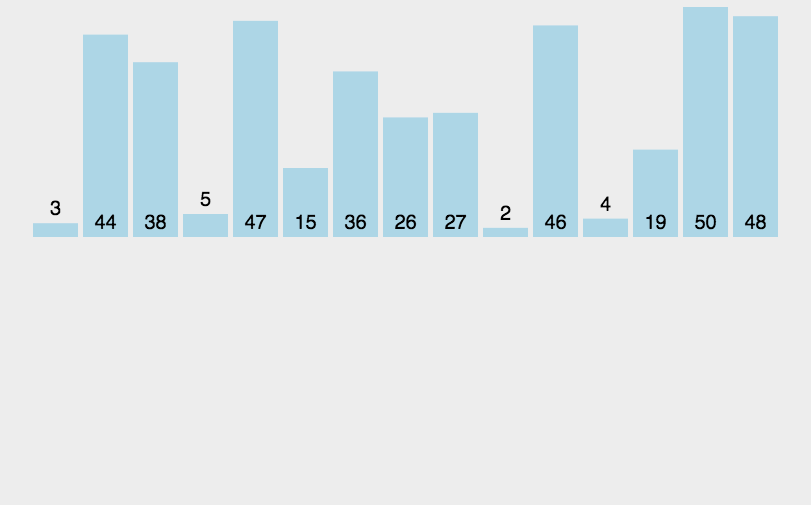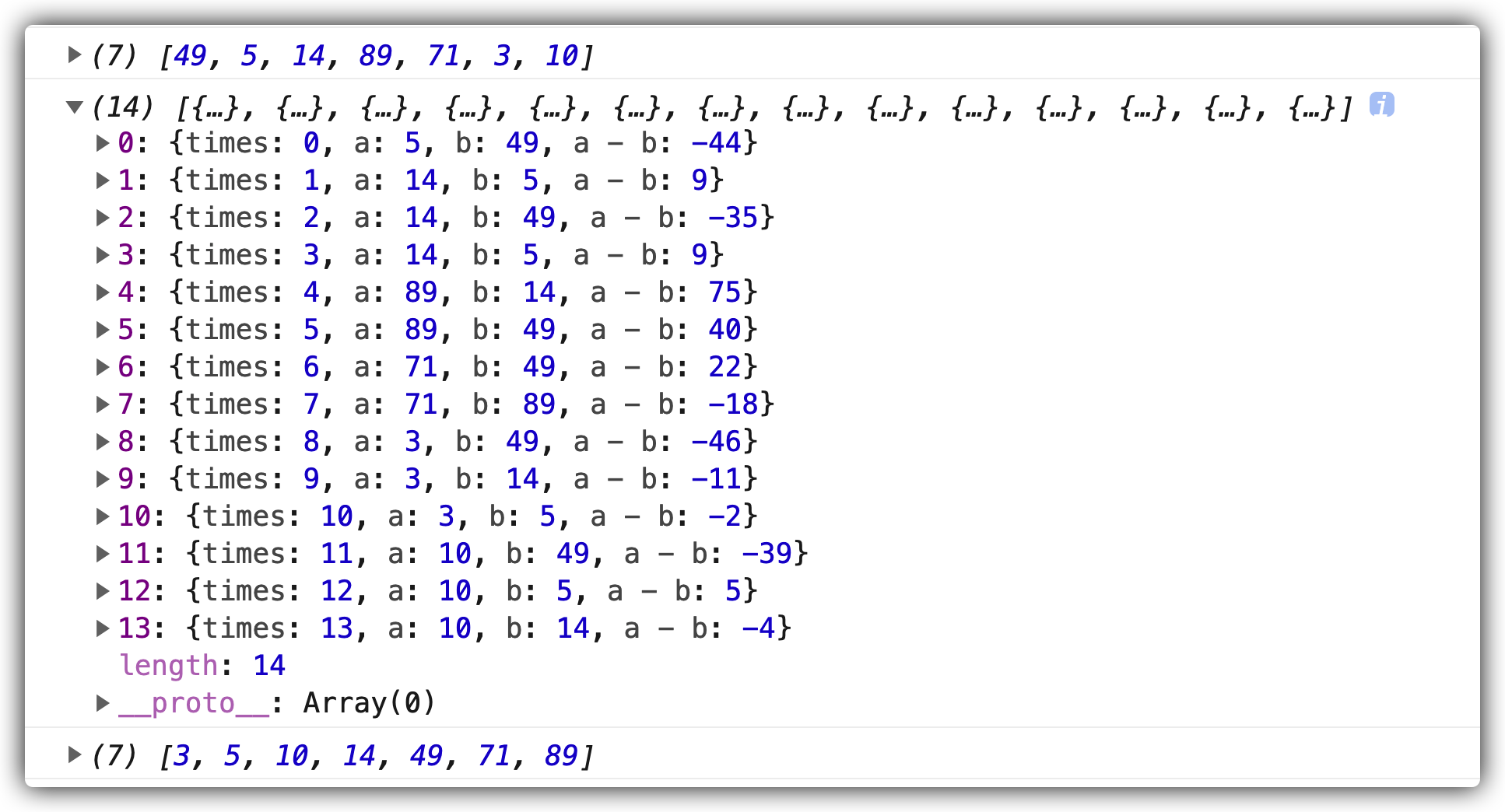# 数组的 sort() 方法详解const arr = [49, 5, 14, 89, 71, 3, 10];// 一般写法arr.sort(function (a, b) {    return a - b;   // 按照升序排列 });// 箭头函数arr.sort((a, b) => a - b);// 结果  [3, 5, 10, 14, 49, 71, 89]

## 插入排序

• 从第一个元素开始，该元素可以认为已经被排序；

• 取出下一个元素，在已经排序的元素序列中从后向前扫描；

• 如果该元素（已排序）大于新元素，将该元素移到下一位置；

• 重复步骤 3，直到找到已排序的元素小于或者等于新元素的位置；

• 将新元素插入到该位置后；

• 重复步骤 2~5。## 关于比较函数 compareFunction

• 如果 compareFunction(a, b) 小于 0 ，那么 a 会被排列到 b 之前。

• 如果 compareFunction(a, b) 等于 0 ， a 和 b 的相对位置不变。

• 如果 compareFunction(a, b) 大于 0 ， b 会被排列到 a 之前。

• compareFunction(a, b) 必须总是对相同的输入返回相同的比较结果，否则排序的结果将是不确定的。

<img src="https://gitee.com/yinyiwang/blogImages/raw/master/images/20210608%20/15-48-16-WecTrT.jpg" alt="question-mark-2318030_1280" style="zoom:50%;" />

## compareFunction 初步探究

const arr = [49, 5, 14, 89, 71, 3, 10];console.log(arr);let times = 0;let res = [];arr.sort((a, b) => {    res.push({times, a, b, "a - b": a - b});    times++;    return a - b;});console.log(res);console.log(arr);• a 的值在按照原数组中的顺序依次变化，这个排序采用的应该是插入排序法

• a 代表的应该是游标

• 从 b 值的变化上又可以看出，a 首次找位置时采用了二分法，a < b 则向前比较， a>b 则向后比较。

### compareFunction 深入探究

function InnerArraySort(array, length, comparefn) {    // In-place QuickSort algorithm.    // For short (length <= 22) arrays, insertion sort is used for efficiency.    if (!IS_CALLABLE(comparefn)) {        comparefn = function (x, y) {            if (x === y) return 0;            if (% _IsSmi(x) && % _IsSmi(y)) {                return % SmiLexicographicCompare(x, y);            }            // 将数组元素转换为字符串            x = TO_STRING(x);            y = TO_STRING(y);            if (x == y) return 0;            else return x < y ? -1 : 1;        };    }    var InsertionSort = function InsertionSort(a, from, to) {        for (var i = from + 1; i < to; i++) {            var element = a[i];            for (var j = i - 1; j >= from; j--) {                var tmp = a[j];                // 调用比较函数 a: tmp, b: element                var order = comparefn(tmp, element);                if (order > 0) {                    a[j + 1] = tmp;                } else {                    break;                }            }            a[j + 1] = element;        }    };    /*** some code here **/     var QuickSort = function QuickSort(a, from, to) {        /*** some code here **/     };}function ArraySort(comparefn) {    CHECK_OBJECT_COERCIBLE(this, "Array.prototype.sort");    var array = TO_OBJECT(this);    var length = TO_LENGTH(array.length);    return InnerArraySort(array, length, comparefn);}// 源码我就不放了，大家有兴趣研究的话，可以点击上面的链接自行查看

1. v8 中实现 sort() 方法时，采用了 ”插入排序“ 和 ”快速排序“ 两种排序方式。

2. 对于短数组（长度 <= 22）来说，插入排序效率更高。

3. 如果没有传入 comparefn ，则生成一个 comparefn 比较函数。

4. 在自动生成的比较函数中，会将传入的数组元素通过 TO_STRING 方法转换为字符串，再行比较。

5. 对比比较函数中的 b 为游标值，这一点和最新版的 chrome 浏览器表现不同。

var order = comparefn(tmp, element);

if (order > 0) {    a[j + 1] = tmp;} else {    break;}

• 如果返回值（a-b）大于 0，即 a > b, 则将当前拿来比较的值 a 复制给它的下一位，并继续使用游标值 b 向前进行比较。

• 如果返回值小于等于 0 ，则结束比较，并将游标值 b 填在最后一次比较值 a 的后面。

## 总结

arr.sort((a,b) => {    const res = a - b;    return res > 0 ? 1 : (res < 0 ? -1 : 0 );});

~本文完## 评论